# Zeta-function method for regularization

(diff) ← Older revision | Latest revision (diff) | Newer revision → (diff)

zeta-function regularization

Regularization and renormalization procedures are essential issues in contemporary physics — without which it would simply not exist, at least in the form known today (2000). They are also essential in supersymmetry calculations. Among the different methods, zeta-function regularization — which is obtained by analytic continuation in the complex plane of the zeta-function of the relevant physical operator in each case — might well be the most beautiful of all. Use of this method yields, for instance, the vacuum energy corresponding to a quantum physical system (with constraints of any kind, in principle). Assuming the corresponding Hamiltonian operator,, has a spectral decomposition of the form (think, as simplest case, of a quantum harmonic oscillator):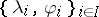, withsome set of indices (which can be discrete, continuous, mixed, multiple, etc.), then the quantum vacuum energy is obtained as follows [a5], [a6]: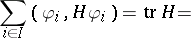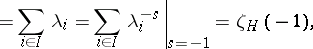where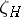is the zeta-function corresponding to the operator. The formal sum over the eigenvalues is usually ill-defined, and the last step involves analytic continuation, inherent to the definition of the zeta-function itself. These mathematically simple-looking relations involve very deep physical concepts (no wonder that understanding them took several decades in the recent history of quantum field theory, QFT). The zeta-function method is unchallenged at the one-loop level, where it is rigorously defined and where many calculations of QFT reduce basically (from a mathematical point of view) to the computation of determinants of elliptic pseudo-differential operators (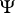DOs, cf. also Pseudo-differential operator) [a2]. It is thus no surprise that the preferred definition of determinant for such operators is obtained through the corresponding zeta-function.

When one comes to specific calculations, the zeta-function regularization method relies on the existence of simple formulas for obtaining the analytic continuation above. These consist of the reflection formula of the corresponding zeta-function in each case, together with some other fundamental expressions, as the Jacobi theta-function identity, Poisson's resummation formula and the famous Chowla–Selberg formula [a2]. However, some of these formulas are restricted to very specific zeta-functions, and it often turned out that for some physically important cases the corresponding formulas did not exist in the literature. This has required a painful process (it has taken over a decade already) of generalization of previous results and derivation of new expressions of this kind [a5], [a6]. [a1].

How to Cite This Entry:
Zeta-function method for regularization. Encyclopedia of Mathematics. URL: http://encyclopediaofmath.org/index.php?title=Zeta-function_method_for_regularization&oldid=13101
This article was adapted from an original article by E. Elizalde (originator), which appeared in Encyclopedia of Mathematics - ISBN 1402006098. See original article